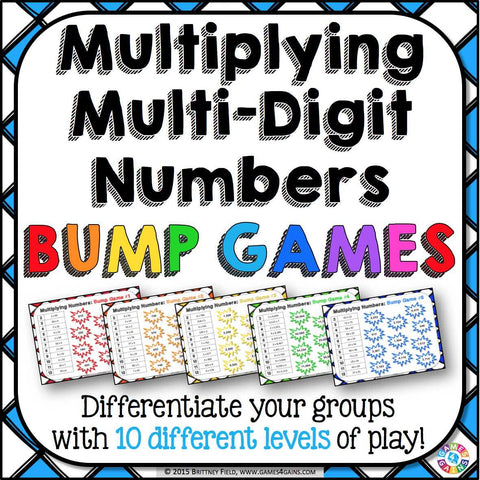## Multiplication Bump Games

• \$450

Multiplication Bump Games contains 10 different multiplication games to help students practice multiplying multi-digit whole numbers.

As students work through these multiplication games, each one ramps up in difficulty (see the image to see all skills covered). This means that you can have all of your students working at their appropriate level when using this set!

Plus, these bump games are so simple to use, and take a minimal amount of prep. Simply print out the game sheet, get 2 dice and 20 counters, and you'll be ready to go!

To play, students will roll the dice and find the corresponding multiplication problem. Then, they have to find the correct product on the game board and cover it with their counter. Students have to get all of the counters onto the game board without getting "bumped" off by the other player.

Included with this Multiplying Multi-Digit Whole Numbers Bump Games set:
Instructions for play
10 different multiplication bump games of varying levels of difficulty, from multiplying two-digit number by one-digit numbers to multiplying four-digit numbers by three-digit numbers